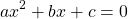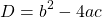# Chapter 7

## Chapter 7 Code Examples

### MATLAB Code Examples

```format bank
bill=input('Enter the amount of the bill (in dollars): ');
if (bill <= 10)
tip = 1.8;
elseif (bill > 10) & (bill <= 60)
tip = bill*0.18;
else
tip = bill*0.2;
end
disp('The tip is (in dollars):')
disp(tip)```
```n = 1;
while (n ~= 0)
x = input (‘Resistance: ‘);
switch x
case 100
disp (x)
case 200
disp (x)
case 300
disp (x)
case 400
disp (x)
otherwise
n = 0;
end
end```

### Python Code Examples

```bill=int(input('Enter the amount of the bill (in dollars): '))
if (bill <= 10):
tip=1.8
elif (bill>10)and(bill<=60):
tip=bill*0.18
else:
tip=bill*0.2
print('The tip is (in dollars): %0.2f'% (tip))```

## Exercises

1. Expanding on problem 1 from chapter 6, implement your algorithm. The problem description is restated below.
Write a script called “quadroots” to solve the real roots of a quadratic equation:Your algorithm should ask the user for the coefficients. To calculate the roots of the equation, your algorithm should calculate the discriminant D given by:If D > 0, the algorithm displays a message: “The equation has two roots” and then displays the roots.
If D = 0, the algorithm displays a message: “The equation has one root”, and then displays the root.
If D < 0, the algorithm displays a message: “The equation has no real roots”.
2. Expanding on problem 2 from chapter 6, implement your algorithm. The problem description is restated below.
Write a program that calculates the cost of a car rental according to the following price schedule:

The program asks the user to enter the rental period and type of car. The program then displays the appropriate cost. If a period longer than 60 days is entered, a message “Rental is not available for more than 60 days” is displayed. If a rental period of less than 6 days is entered for Class D, a message “Class D cars cannot be rented for less than 6 days” is displayed.